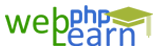Apply for Zend Framework Certification Training

# Javascript

```Hi viewers
using javascript we can create a simple calculator.
to perform this calculation we must know some of the mathematical
function to do calculations.
While Doing Calculation Work in JavaScript

we use following functions
var price = \$("#id").val();
1)    parseInt(price);        //If you want to convert into integer format
2)    parseFloat(price);    //If you want to convert into Float Format
3)    price.toFixed(2);       //Display Decimal up to 2 place

```

`{{questionlistdata.blog_question_description}}`
`{{answer.blog_answer_description  }}`